# Building a Generative Adversarial Network using Keras

This article will demonstrate how to build a Generative Adversarial Network using the Keras library. The dataset which is used is the CIFAR10 Image dataset which is preloaded into Keras. You can read about the dataset here.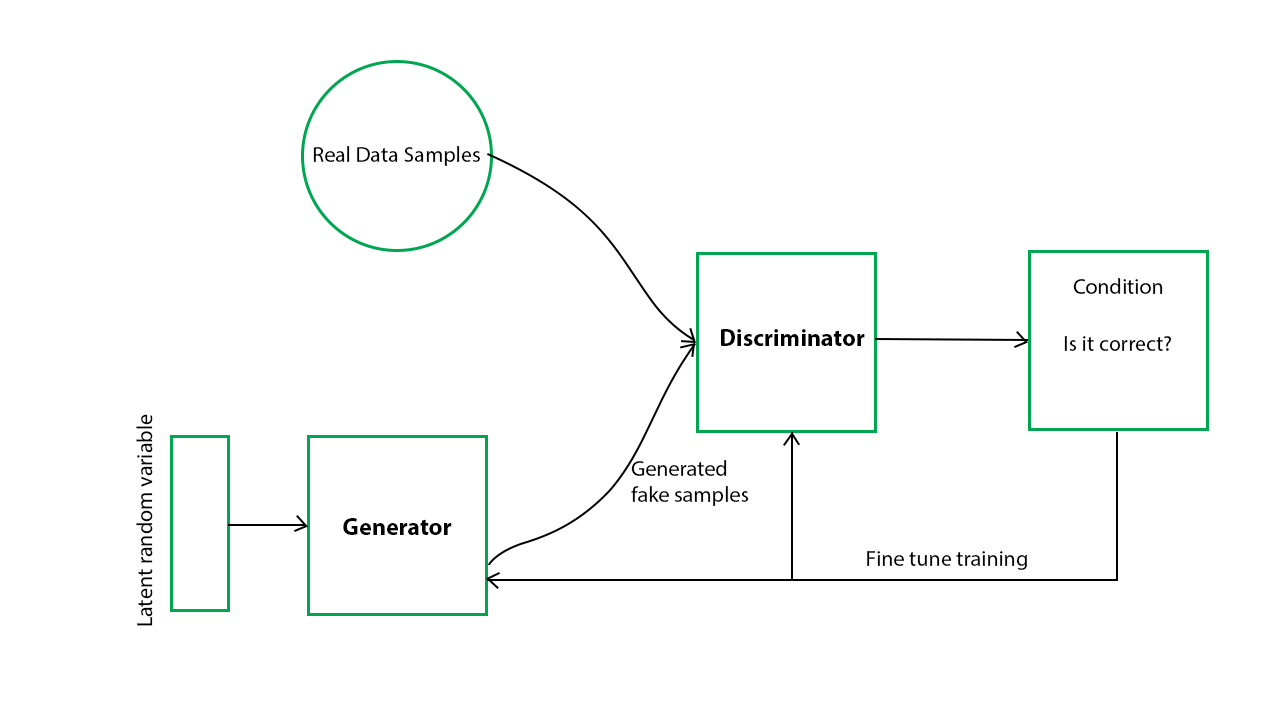Step 1: Importing the required libraries

 `import` `numpy as np ` `import` `matplotlib.pyplot as plt ` `import` `keras ` `from` `keras.layers ``import` `Input``, Dense, Reshape, Flatten, Dropout ` `from` `keras.layers ``import` `BatchNormalization, Activation, ZeroPadding2D ` `from` `keras.layers.advanced_activations ``import` `LeakyReLU ` `from` `keras.layers.convolutional ``import` `UpSampling2D, Conv2D ` `from` `keras.models ``import` `Sequential, Model ` `from` `keras.optimizers ``import` `Adam,SGD `

 `#Loading the CIFAR10 data ` `(X, y), (_, _) ``=` `keras.datasets.cifar10.load_data() ` ` `  `#Selecting a single class images ` `#The number was randomly chosen and any number ` `#between 1 to 10 can be chosen ` `X ``=` `X[y.flatten() ``=``=` `8``] `

Step 3: Defining parameters to be used in later processes

 `#Defining the Input shape ` `image_shape ``=` `(``32``, ``32``, ``3``) ` `         `  `latent_dimensions ``=` `100`

Step 4: Defining a utility function to build the Generator

 `def` `build_generator(): ` ` `  `        ``model ``=` `Sequential() ` ` `  `        ``#Building the input layer ` `        ``model.add(Dense(``128` `*` `8` `*` `8``, activation``=``"relu"``, ` `                        ``input_dim``=``latent_dimensions)) ` `        ``model.add(Reshape((``8``, ``8``, ``128``))) ` `         `  `        ``model.add(UpSampling2D()) ` `         `  `        ``model.add(Conv2D(``128``, kernel_size``=``3``, padding``=``"same"``)) ` `        ``model.add(BatchNormalization(momentum``=``0.78``)) ` `        ``model.add(Activation(``"relu"``)) ` `         `  `        ``model.add(UpSampling2D()) ` `         `  `        ``model.add(Conv2D(``64``, kernel_size``=``3``, padding``=``"same"``)) ` `        ``model.add(BatchNormalization(momentum``=``0.78``)) ` `        ``model.add(Activation(``"relu"``)) ` `         `  `        ``model.add(Conv2D(``3``, kernel_size``=``3``, padding``=``"same"``)) ` `        ``model.add(Activation(``"tanh"``)) ` ` `  ` `  `        ``#Generating the output image ` `        ``noise ``=` `Input``(shape``=``(latent_dimensions,)) ` `        ``image ``=` `model(noise) ` ` `  `        ``return` `Model(noise, image) `

Step 5: Defining a utility function to build the Discriminator

 `def` `build_discriminator(): ` ` `  `        ``#Building the convolutional layers ` `        ``#to classify whether an image is real or fake ` `        ``model ``=` `Sequential() ` ` `  `        ``model.add(Conv2D(``32``, kernel_size``=``3``, strides``=``2``, ` `                         ``input_shape``=``image_shape, padding``=``"same"``)) ` `        ``model.add(LeakyReLU(alpha``=``0.2``)) ` `        ``model.add(Dropout(``0.25``)) ` `         `  `        ``model.add(Conv2D(``64``, kernel_size``=``3``, strides``=``2``, padding``=``"same"``)) ` `        ``model.add(ZeroPadding2D(padding``=``((``0``,``1``),(``0``,``1``)))) ` `        ``model.add(BatchNormalization(momentum``=``0.82``)) ` `        ``model.add(LeakyReLU(alpha``=``0.25``)) ` `        ``model.add(Dropout(``0.25``)) ` `         `  `        ``model.add(Conv2D(``128``, kernel_size``=``3``, strides``=``2``, padding``=``"same"``)) ` `        ``model.add(BatchNormalization(momentum``=``0.82``)) ` `        ``model.add(LeakyReLU(alpha``=``0.2``)) ` `        ``model.add(Dropout(``0.25``)) ` `         `  `        ``model.add(Conv2D(``256``, kernel_size``=``3``, strides``=``1``, padding``=``"same"``)) ` `        ``model.add(BatchNormalization(momentum``=``0.8``)) ` `        ``model.add(LeakyReLU(alpha``=``0.25``)) ` `        ``model.add(Dropout(``0.25``)) ` `         `  `        ``#Building the output layer ` `        ``model.add(Flatten()) ` `        ``model.add(Dense(``1``, activation``=``'sigmoid'``)) ` ` `  `        ``image ``=` `Input``(shape``=``image_shape) ` `        ``validity ``=` `model(image) ` ` `  `        ``return` `Model(image, validity) `

Step 6: Defining a utility function to display the generated images

 `def` `display_images(): ` `        ``r, c ``=` `4``,``4` `        ``noise ``=` `np.random.normal(``0``, ``1``, (r ``*` `c,latent_dimensions)) ` `        ``generated_images ``=` `generator.predict(noise) ` ` `  `        ``#Scaling the generated images ` `        ``generated_images ``=` `0.5` `*` `generated_images ``+` `0.5` ` `  `        ``fig, axs ``=` `plt.subplots(r, c) ` `        ``count ``=` `0` `        ``for` `i ``in` `range``(r): ` `            ``for` `j ``in` `range``(c): ` `                ``axs[i,j].imshow(generated_images[count, :,:,]) ` `                ``axs[i,j].axis(``'off'``) ` `                ``count ``+``=` `1` `        ``plt.show() ` `        ``plt.close() `

Step 7: Building the Generative Adversarial Network

 `# Building and compiling the discriminator ` `discriminator ``=` `build_discriminator() ` `discriminator.``compile``(loss``=``'binary_crossentropy'``, ` `                      ``optimizer``=``Adam(``0.0002``,``0.5``), ` `                    ``metrics``=``[``'accuracy'``]) ` ` `  `#Making the Discriminator untrainable ` `#so that the generator can learn from fixed gradient ` `discriminator.trainable ``=` `False` ` `  `# Building the generator ` `generator ``=` `build_generator() ` ` `  `#Defining the input for the generator ` `#and generating the images ` `z ``=` `Input``(shape``=``(latent_dimensions,)) ` `image ``=` `generator(z) ` ` `  ` `  `#Checking the validity of the generated image ` `valid ``=` `discriminator(image) ` ` `  `#Defining the combined model of the Generator and the Discriminator ` `combined_network ``=` `Model(z, valid) ` `combined_network.``compile``(loss``=``'binary_crossentropy'``, ` `                         ``optimizer``=``Adam(``0.0002``,``0.5``)) `

Step 8: Training the network

 `num_epochs``=``15000` `batch_size``=``32` `display_interval``=``2500` `losses``=``[] ` ` `  `#Normalizing the input ` `X ``=` `(X ``/` `127.5``) ``-` `1.` `         `  ` `  `#Defining the Adversarial ground truths ` `valid ``=` `np.ones((batch_size, ``1``)) ` ` `  `#Adding some noise  ` `valid ``+``=` `0.05` `*` `np.random.random(valid.shape) ` `fake ``=` `np.zeros((batch_size, ``1``)) ` `fake ``+``=` `0.05` `*` `np.random.random(fake.shape) ` ` `  `for` `epoch ``in` `range``(num_epochs): ` `             `  `            ``#Training the Discriminator ` `             `  `            ``#Sampling a random half of images ` `            ``index ``=` `np.random.randint(``0``, X.shape[``0``], batch_size) ` `            ``images ``=` `X[index] ` ` `  `            ``#Sampling noise and generating a batch of new images ` `            ``noise ``=` `np.random.normal(``0``, ``1``, (batch_size, latent_dimensions)) ` `            ``generated_images ``=` `generator.predict(noise) ` `             `  ` `  `            ``#Training the discriminator to detect more accurately ` `            ``#whether a generated image is real or fake ` `            ``discm_loss_real ``=` `discriminator.train_on_batch(images, valid) ` `            ``discm_loss_fake ``=` `discriminator.train_on_batch(generated_images, fake) ` `            ``discm_loss ``=` `0.5` `*` `np.add(discm_loss_real, discm_loss_fake) ` `             `  `            ``#Training the Generator ` ` `  `            ``#Training the generator to generate images ` `            ``#which pass the authenticity test ` `            ``genr_loss ``=` `combined_network.train_on_batch(noise, valid) ` `             `  `            ``#Tracking the progress                 ` `            ``if` `epoch ``%` `display_interval ``=``=` `0``: ` `                 ``display_images() `

Epoch 0: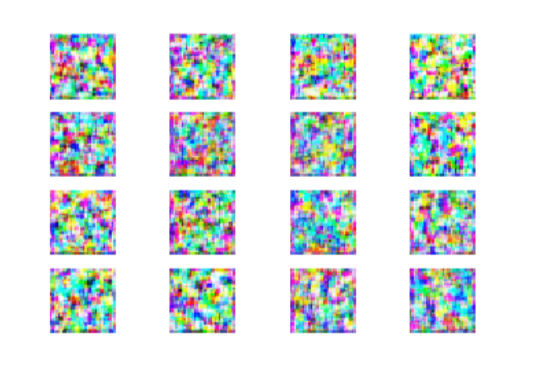Epoch 2500: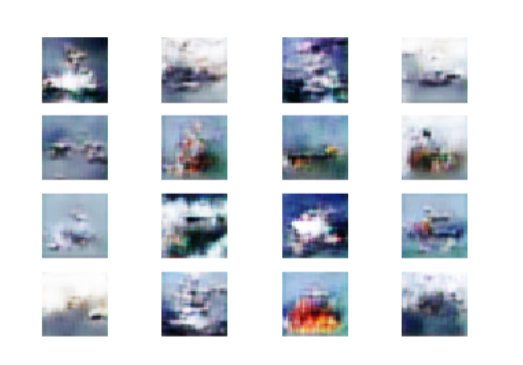Epoch 5000: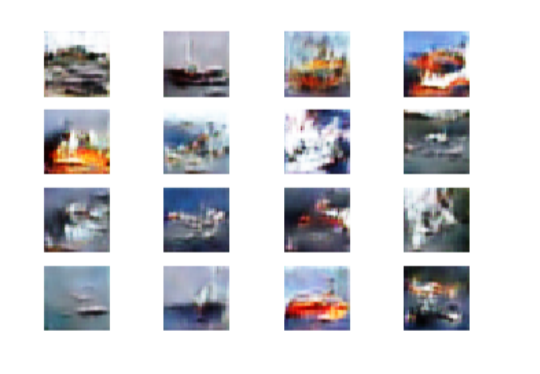Epoch 7500: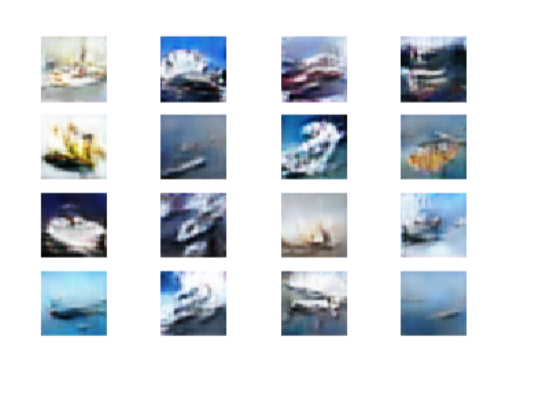Epoch 10000: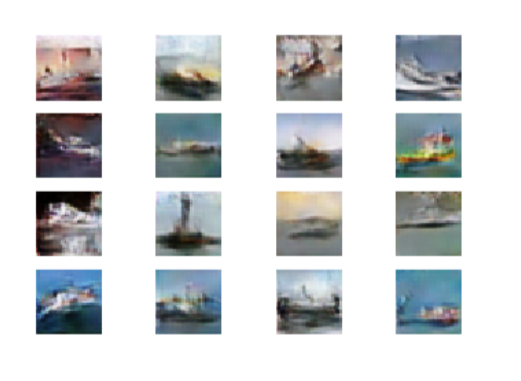Epoch 12500: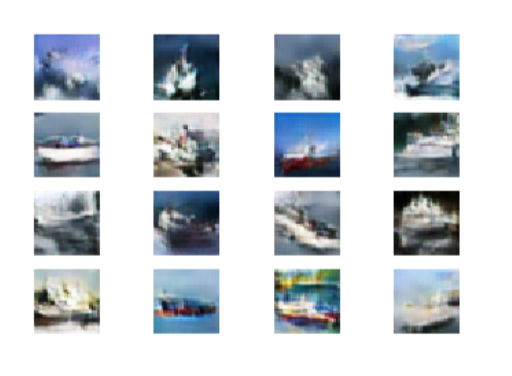Note that the quality of images increases with each epoch.

Step 8: Evaluating the performance

The performance of the network will be evaluated by comparing the images generated on the last epoch to the original images visually.

a) Plotting the original images

 `#Plotting some of the original images  ` `s``=``X[:``40``] ` `s ``=` `0.5` `*` `s ``+` `0.5` `f, ax ``=` `plt.subplots(``5``,``8``, figsize``=``(``16``,``10``)) ` `for` `i, image ``in` `enumerate``(s): ` `    ``ax[i``/``/``8``, i``%``8``].imshow(image) ` `    ``ax[i``/``/``8``, i``%``8``].axis(``'off'``) ` `         `  `plt.show() `b) Plotting the images generated on the last epoch

 `#Plotting some of the last batch of generated images ` `noise ``=` `np.random.normal(size``=``(``40``, latent_dimensions)) ` `generated_images ``=` `generator.predict(noise) ` `generated_images ``=` `0.5` `*` `generated_images ``+` `0.5` `f, ax ``=` `plt.subplots(``5``,``8``, figsize``=``(``16``,``10``)) ` `for` `i, image ``in` `enumerate``(generated_images): ` `    ``ax[i``/``/``8``, i``%``8``].imshow(image) ` `    ``ax[i``/``/``8``, i``%``8``].axis(``'off'``) ` `         `  `plt.show() `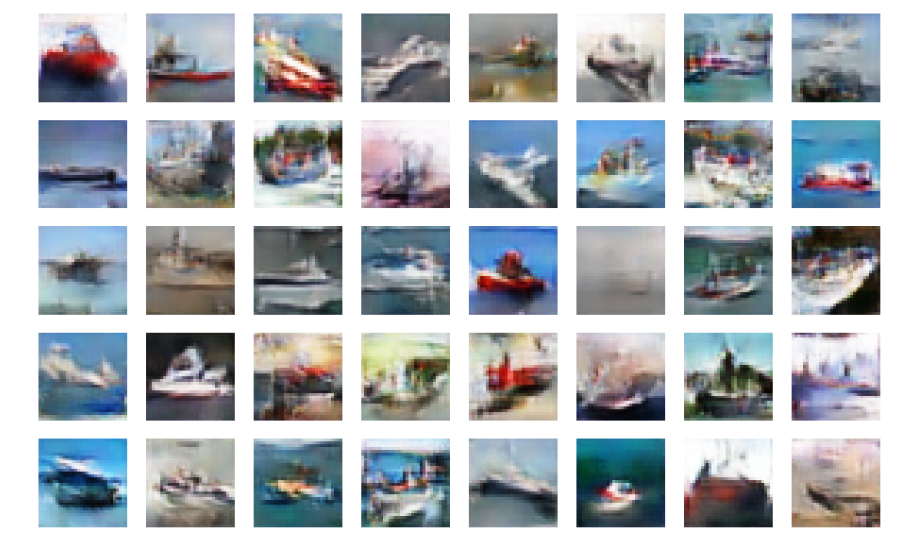On visually comparing the two sets of images, it can be concluded that the network is working at an acceptable level. The quality of images can be improved by training the network for more time or by tuning the parameters of the network.

My Personal Notes arrow_drop_upCheck out this Author's contributed articles.

If you like GeeksforGeeks and would like to contribute, you can also write an article using contribute.geeksforgeeks.org or mail your article to contribute@geeksforgeeks.org. See your article appearing on the GeeksforGeeks main page and help other Geeks.

Please Improve this article if you find anything incorrect by clicking on the "Improve Article" button below.

Article Tags :
Practice Tags :

Be the First to upvote.

Please write to us at contribute@geeksforgeeks.org to report any issue with the above content.